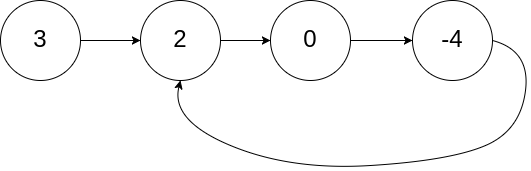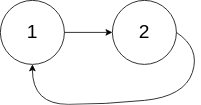Given a linked list, determine if it has a cycle in it.

To represent a cycle in the given linked list, we use an integer `pos` which represents the position (0-indexed) in the linked list where tail connects to. If `pos` is `-1`, then there is no cycle in the linked list.

Example 1:

``````Input: head = [3,2,0,-4], pos = 1
Output: true
Explanation: There is a cycle in the linked list, where tail connects to the second node.
``````Example 2:

``````Input: head = [1,2], pos = 0
Output: true
Explanation: There is a cycle in the linked list, where tail connects to the first node.
``````Example 3:

``````Input: head = , pos = -1
Output: false
Explanation: There is no cycle in the linked list.
``````Can you solve it using  O(1)  (i.e. constant) memory?

C++ 解法：

``````class Solution {
public:
while (fast && fast->next) {
slow = slow->next;
fast = fast->next->next;
if (slow == fast) return true;
}
return false;
}
};
``````

Java 解法：

``````public class Solution {
while (fast != null && fast.next != null) {
slow = slow.next;
fast = fast.next.next;
if (slow == fast) return true;
}
return false;
}
}
``````

Github 同步地址：

https://github.com/grandyang/leetcode/issues/141

Happy Number

 微信打赏Venmo 打赏